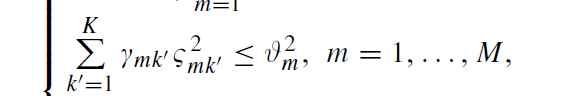# Disciplined convex programming error: Invalid constraint: {convex} <= {convex}

I am new to CVX and i tried the following optimization problem:
I cut out most of the code to keep only the wrong part. I wrote the formula in the picture%% ---------------------------------------------------------------------
cvx_begin
variable rou(K,K)
variable V(M,1)
variable cauy(M,K)
sb2_left = cvx(zeros(M,1));
sb2_right = cvx(zeros(M,1));

%% -----------------------------calculate subect 2 left
for m=1:M
sb2_left(m) = sum(Gamma(m,: ) .* (cauy(m,:).^2));
end
%% -----------------------------calculate subect 2 right
for m=1:M
sb2_right(m) = V(m)^2;
end
%% -----------------------------subject------------------------------
subject to
for m=1:M
sb2_left(m) <= sb2_right(m);
end
for m=1:M
0 <= V(m) <=1;
end
cvx_end
i got the following error message for line 20 in my code, sb2_left(m) <= sb2_right(m) ;:
Disciplined convex programming error: Invalid constraint: {convex} <= {convex}
What’s wrong?
can anyone help me with this problem?

This is addressed in the FAQ.

Thanks for your reply. I read the FAQ based on your suggestion, I think my constraint is not in line with the DCP ruleset. This problem has been proved to be quasi-convex, so to solve some of the feasibility issues. My purpose is to reproduce the original author’s results, how can I modify the constraint expression, can you give me some advice?

I have the same problem. Did you find a solution for it ?

@S.Elsalam If you show your complete reproducible code, perhaps someone can offer advice. But before doing that, please read Why isn’t CVX accepting my model? READ THIS FIRST! and the CVX User’s Guide http://cvxr.com/cvx/doc/

thank you so much. I will read it.

I have read it but I don’t find a solution for the problem I have.
subject to
for iUserIndex = 1 : NumberOfUsers
zeros(1 , NumberOfAPs) <= Mu_New <= ones(1 , NumberOfAPs);
New_Eita >= zeros(NumberOfUsers , NumberOfAPs);
sum(New_Eita.^2 .* ALL_Users_Gamma_k) <= Mu_New.^2;
end
Matlab gives me this error
Error using cvxprob/newcnstr (line 192)
Disciplined convex programming error:
Invalid constraint: {convex} <= {convex}

Actually, this is not my optimization problem. I am just trying to implement the same problem that mentioned above and I got the same error !!

If Mu_New. only appears as shown (and not otherwise in non-squared form), then you can use something along the lines of (this may not be quite right, because you don’t seem to have paid much attention to the indexing, and in any event have not shown us your complete code, including variable declarations):

``````variable Mu_new_squared(NumberOfAPs)
0 <=  Mu_new_squared <= 1
...
sum(New_Eita.^2 .* ALL_Users_Gamma_k) <= Mu_New_squared
``````

Otherwise, the problem is non-convex if Mu_New is a CVX variable.

unfortunately, it appears in non-squared form in other constraints.
sorry for bothering you but I have just started working on optimization and I have one more question that may helps. if its is a slack variable the definition will be changed or it will be the same as other variables ?!.

if its is a slack variable the definition will be changed or it will be the same as other variables ?!.

Is what definition changed? A slack variable is declared the same as any other variable. You can include the `nonnegative` keyword as part of the declaration, or add a constraint to constrain it to be nonnegative.

`variable s nonnegative % slack variable`

ok, I have already added a constraint for it to be positive
is there any other way to write this constraint to solve this problem or not !!

If the variable in question can not be handled only in squared form, then the problem is non-convex, and CVX will not accept it. Please re-read Why isn't CVX accepting my model? READ THIS FIRST! .

Excuse me, I am also looking at this problem recently, have you solved it?

@Li_Guoyu What specifcally is the problem you are trying to solve? Have you proven is is convex? Please read the link in my previous post.

Thank you. I have read.I need to continue thinking.

I get tge same error with following constraint. S(real) and W (complex) are cvx variables

inv_pos(S) <= delta * P * abs(trace(W * H’ * (v*v’) *H ))

Can you help?

Is it convex? It seems not.

@jackfsuia, I was thinking since it can also be written as 1/xy <= e^0 then it is convex for x,y > 0

but w is complex, and abs() is put on it in your post. if abs() was changed to real(), that might be good.

Thank you @jackfsuia , I see now. It works after replacing abs() with real().But, won’t throwing away imaginary component affect the solution?